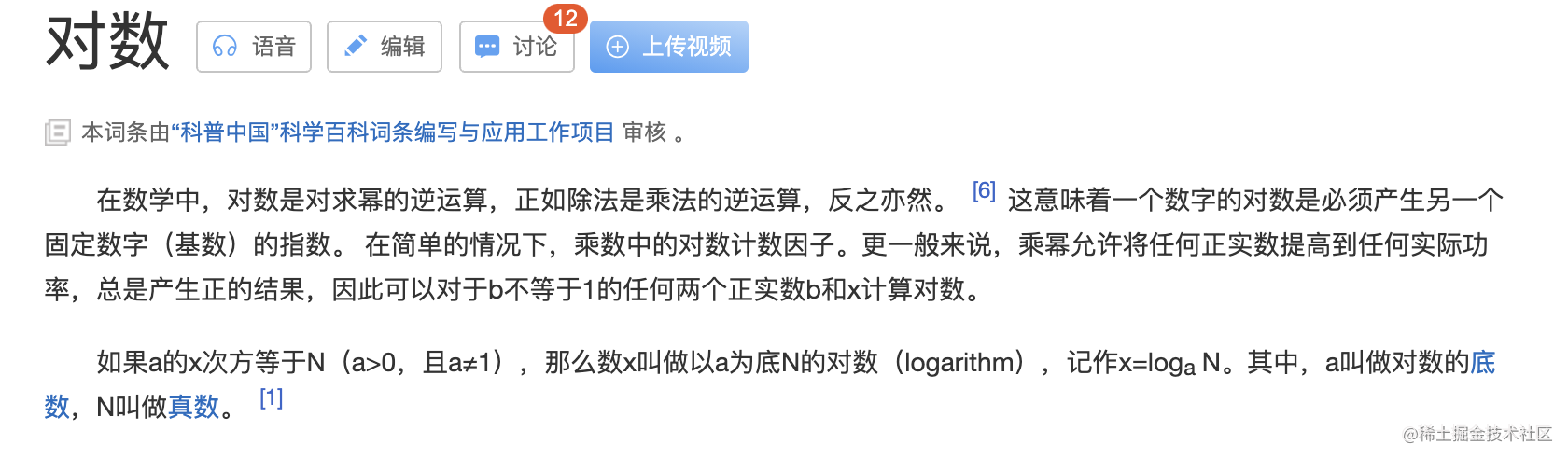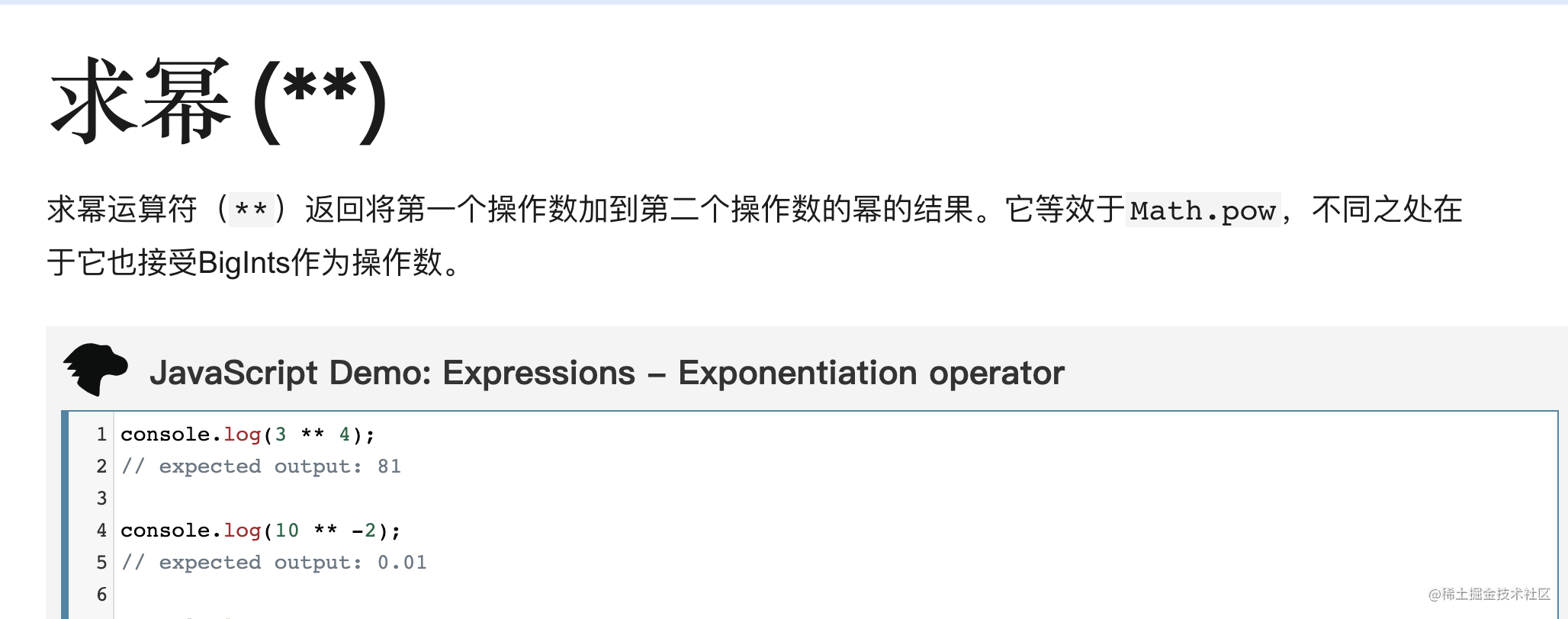# 【算法】 时间复杂度，空间复杂度

## 一、时间复杂度

##### 1） 常数阶O(1)

``````int i = 1;
int j = 2;
++i;
j++;
int m = i + j;

##### 2) 线性阶O(n)
``````for(i=1; i<=n; ++i)
{
j = i;
j++;
}

##### 3) 对数阶O(logN)``````int i = 1;
while(i<n)
{
i = i * 2;
}

##### 4) 线性对数阶O(nlogN)

``````for(m=1; m<n; m++)
{
i = 1;
while(i<n)
{
i = i * 2;
}
}

##### 5) 平方阶O(n²)

``````for(x=1; i<=n; x++)
{
for(i=1; i<=n; i++)
{
j = i;
j++;
}
}

``````
for(x=1; i<=m; x++)
{
for(i=1; i<=n; i++)
{
j = i;
j++;
}
}